# Search

About 58 Search Results Matching Types of Worksheet, Worksheet Section, Generator, Generator Section, Grades matching 5th Grade, Similar to Reading a Protractor Worksheet 1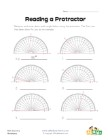## Reading a Protractor Worksheet 1

Read each protractor and write down the angles the...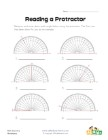## Reading a Protractor Worksheet 2

Read each protractor and write down the angles the...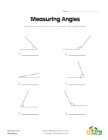## Measuring Angles Worksheet 1

Measure each of the angles with a protractor and w...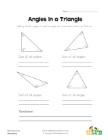## Angles in a Triangle Worksheet

Kids learn that the angles of a triangle always ad...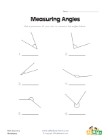## Measuring Angles Worksheet 2

Use a protractor to measure each of the angles.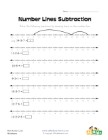## Number Lines Subtraction Worksheet 1

Use the number lines to solve the subtraction prob...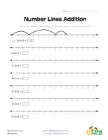## Number Lines Addition Worksheet 1

Use the number lines to solve the addition problem...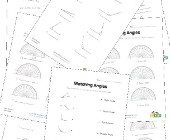## Angles Worksheets

Help kids learn about angles with this collection ...